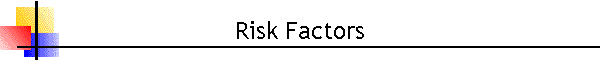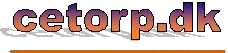Up • Introduction • My profile • Services • Nordtest • GumXp • Risk Factors • CCL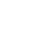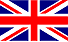Se denne side på Dansk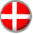## A spreadsheet for Calculation of Risk Factors

See the description of file formats and macros at the bottom of this page.

- Use the left mouse button to open the file in a window.

- Use the right mouse button to save the file to harddisk.

The Calculation of Risk Factor is a spreadsheet program used to calculate risk factors for selected subsystems within larger IT-systems. The higher the risk factor calculated for a given subsystem is, the greater will the threat that this subsystem poses to the company be, and the greater will the need for preventive actions by means of proper software validation of the subsystem be. The calculated risk factors should only be used in this context and are not meant as a general measure for risks caused by software malfunction and errors. They are simply meant as a mean to point out those software subsystems that most certainly require validation.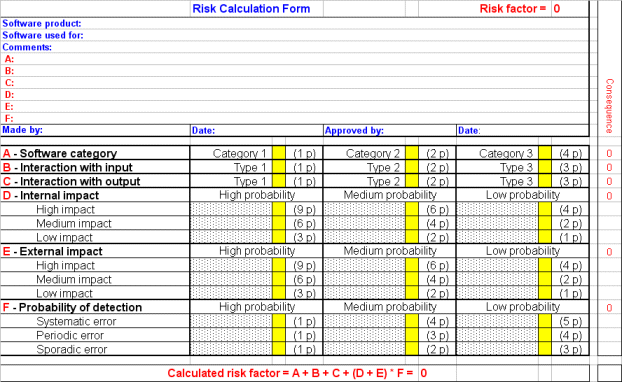The Calculation of Risk Factor calculates risk factors (value 0 - 100) by adding the weighted marks in the spreadsheet cells. The risk factor is a relative quantity which only has meaning when compared to other factors estimated in the system perspective (i.e. estimated relative to the actual system, not a particular process in the system). All sheets use the same score values which are located in a hidden sheet "Basis" - if the score values in here are changed, the calculations on all other sheets will change accordingly. The sheet "Template" is meant as an empty form that can be duplicated by means of the Excel function "Move or Copy Sheet...".

The Calculation of Risk Factor features:

• Calculation of Risk Factor uses approved risk assessment methods
• Almost unlimited number of calculation sheets in a workbook
• New risk calculations are based on the (changeable) template "Template"
• The score system is common to all sheets and are found on the hidden sheet "Basis"
• Pre-programmed settings ...
• Cells for cross-marks  x  are yellow (avoid space as mark character)
• Remaining cells except the upper area for comments are write-protected
• The score value is shown in brackets as a link to the corresponding cell in "Basis"
• Double-marks are automatically indicated as Error
• Macro-codes for calculation reside in a protected "Module1" in the Visual Basic Editor ...
• The product is free of charge - the VBA password is "cet", however ...
• ... keep the password to prevent inadvertently change of the code
• No restriction in using user-developed macros
• Calculation of Risk Factor is in English

The Calculation of Risk Factor software package contains:

 Calculation of Risk Factor. PDF PDF document, description and guidelines Calculation of Risk Factor.XLS Excel spreadsheet, example and template F26 Temp ITS90 - Risk Factor.XLS Excel spreadsheet, example and maximum score

The Calculation of Risk Factor software package can be downloaded free of charge. It is the user's responsibility that the product is used in accordance with the intentions mentioned above. The product is freeware and the user may freely modify the spreadsheet as required.

### File formats and macros

```ZIP    	Compressed file
May require WinZip® unpack program www.winzip.com (depends on the operative system)```
```PDF	Adobe Portable Document Format file
```XLS    	Excel spreadsheet file